# Calculus 3 : Absolute Minimums and Maximums

## Example Questions

### Example Question #1 : Absolute Minimums And Maximums

Find the absolute minimums and maximums of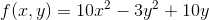on the disk of radius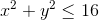.

Absolute Minimum: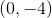Absolute Maximum: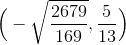Absolute Minimum:Absolute Maximum: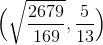Absolute Minimum:Absolute Maximum:Absolute Minimum: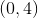Absolute Maximum:Absolute Minimum:Absolute Maximum:Absolute Minimum:Absolute Maximum:Explanation:

The first thing we need to do is find the partial derivative in respect to, and.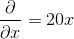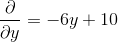We need to find the critical points, so we set each of the partials equal to.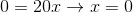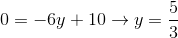We only have one critical point at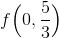, now we need to find the function value in order to see if it is inside or outside the disk.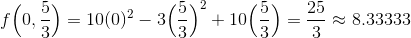This is within our disk.

We now need to take a look at the boundary,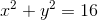. We can solve for, and plug it into.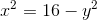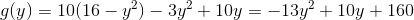We will need to find the absolute extrema of this function on the range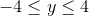. We need to find the critical points of this function.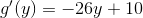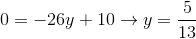The function value at the critical points and end points are: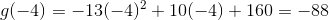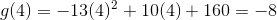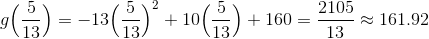Now we need to figure out the values ofthese correspond to.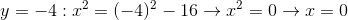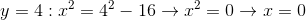Now lets summarize our results as follows: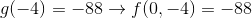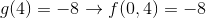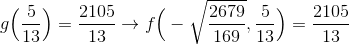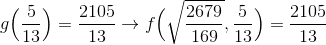From this we can conclude that there is an absolute minimum at, and two absolute maximums atand.